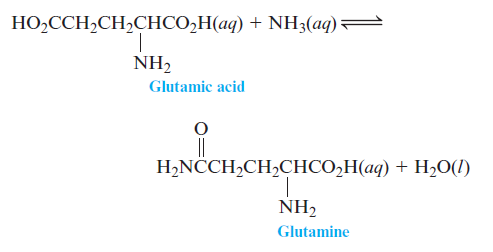# Problem: Exercise 75. Cells use the hydrolysis of adenosine triphosphate, abbreviated as ATP, as a source of energy. Symbolically, this reaction can be written asATP(aq) + H2O(l) → ADP(aq) + H2PO4-(aq)where ADP represents adenosine diphosphate. For this reaction, ΔG° = —30.5 kJ/mol.a. Calculate K at 25°C.b. If all the free energy from the metabolism of glucoseC6H12O6(s) + 6O2(g) → 6CO2(g) + 6H2O(l)goes into forming ATP from ADP, how many ATP molecules can be produced for every molecule of glucose?One reaction that occurs in human metabolism is

###### FREE Expert Solution

a. Recall that ΔG˚rxn and K are related to each other:

K = 2.20x105

b. Calculate Δ°G

90% (318 ratings)###### Problem Details

Exercise 75. Cells use the hydrolysis of adenosine triphosphate, abbreviated as ATP, as a source of energy. Symbolically, this reaction can be written as

ATP(aq) + H2O(l) → ADP(aq) + H2PO4-(aq)

where ADP represents adenosine diphosphate. For this reaction, ΔG° = —30.5 kJ/mol.

a. Calculate K at 25°C.
b. If all the free energy from the metabolism of glucose

C6H12O6(s) + 6O2(g) → 6CO2(g) + 6H2O(l)

goes into forming ATP from ADP, how many ATP molecules can be produced for every molecule of glucose?

One reaction that occurs in human metabolism isWhat scientific concept do you need to know in order to solve this problem?

Our tutors have indicated that to solve this problem you will need to apply the Gibbs Free Energy concept. You can view video lessons to learn Gibbs Free Energy. Or if you need more Gibbs Free Energy practice, you can also practice Gibbs Free Energy practice problems.

What is the difficulty of this problem?

Our tutors rated the difficulty ofExercise 75. Cells use the hydrolysis of adenosine triphosph...as high difficulty.

What professor is this problem relevant for?

Based on our data, we think this problem is relevant for Professor Weiss' class at UCSC.

What textbook is this problem found in?

Our data indicates that this problem or a close variation was asked in Chemistry: An Atoms First Approach - Zumdahl Atoms 1st 2nd Edition. You can also practice Chemistry: An Atoms First Approach - Zumdahl Atoms 1st 2nd Edition practice problems.